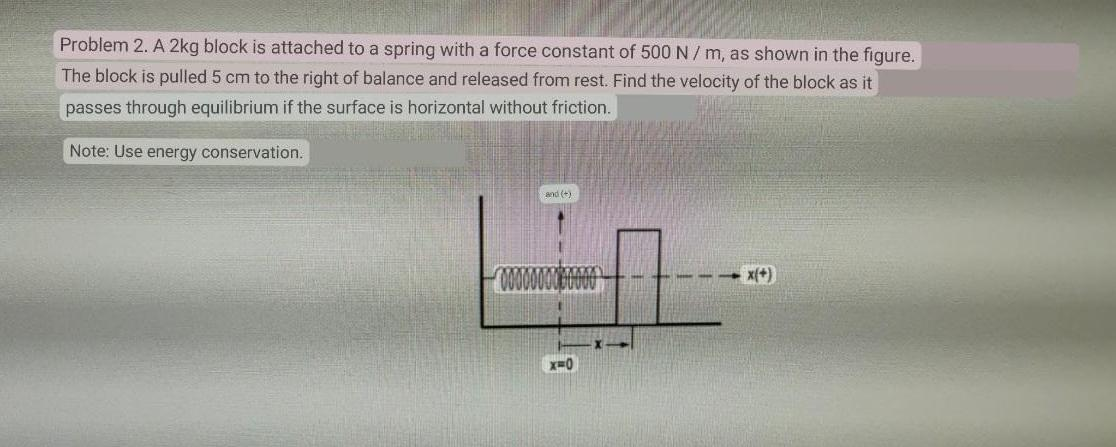# Question Solved1 AnswerProblem 2. A 2kg block is attached to a spring with a force constant of 500 N/m, as shown in the figure. The block is pulled 5 cm to the right of balance and released from rest. Find the velocity of the block as it passes through equilibrium if the surface is horizontal without friction. Note: Use energy conservation. and x(+) X=0Transcribed Image Text: Problem 2. A 2kg block is attached to a spring with a force constant of 500 N/m, as shown in the figure. The block is pulled 5 cm to the right of balance and released from rest. Find the velocity of the block as it passes through equilibrium if the surface is horizontal without friction. Note: Use energy conservation. and x(+) X=0
More
Transcribed Image Text: Problem 2. A 2kg block is attached to a spring with a force constant of 500 N/m, as shown in the figure. The block is pulled 5 cm to the right of balance and released from rest. Find the velocity of the block as it passes through equilibrium if the surface is horizontal without friction. Note: Use energy conservation. and x(+) X=0